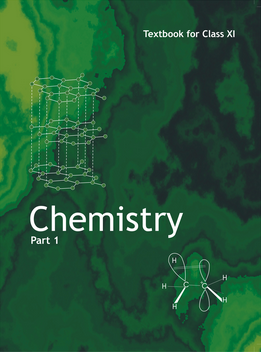# Basic concepts of Chemistry (Weightage 2%)   Share

### Topics from Basic concepts of Chemistry

• Chemical reaction (2 concepts)
• General introduction : Important and scope of chemistry (8 concepts)
• Atomic and molecular masses (1 concepts)
• Mole concept and molar masses (1 concepts)
• Percentage composition and & empirical and molecular formula (3 concepts)
• Stoichiometry and calculations based on stoichiometry (2 concepts)
• Precision and accuracy, significant figures, S.I. Units, dimensional analysis (2 concepts)
• Matter and its nature (2 concepts)
• Dalton's atomic theory (4 concepts)
• Laws of chemical combination (4 concepts)
• Atomic and molecular masses, mole concept, molar mass (3 concepts)
• Importance of chemistry and Nature of matter (2 concepts)
• Physical quantities and their measurements in chemistry (1 concepts)
• Volume Strength of H2O2 Solution and Hardness of Water (1 concepts)
• n-Factor or Valence Factor (1 concepts)
• Uncertainty in measurement (1 concepts)
• Fundamental Laws of Chemical combinations (1 concepts)
• Percentage Composition (2 concepts)
• Dalton’s Atomic Model (1 concepts)
• Atomic And Molecular Masses (1 concepts)
• Mole Concept And Molar Masses (1 concepts)
• Stoichiometry, Stoichiometric Calculations (3 concepts)
• Smart Tips: Some Basic Concept in Chemistry (1 concepts)

### Important Books for Basic concepts of Chemistry

•Exams
Articles
Questions